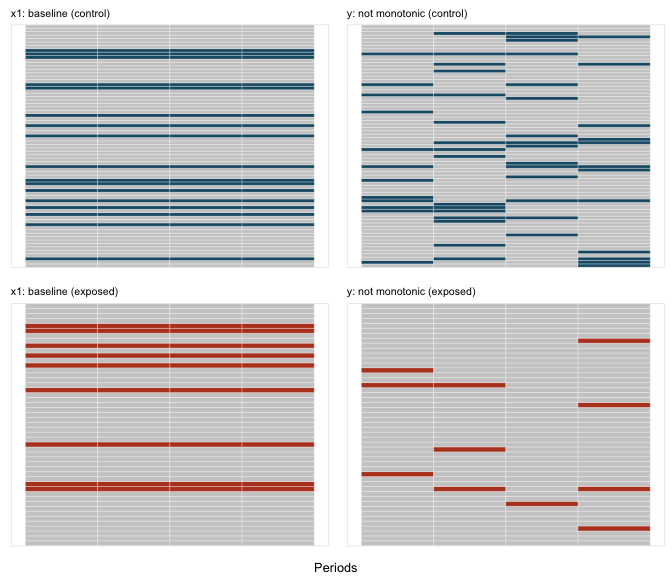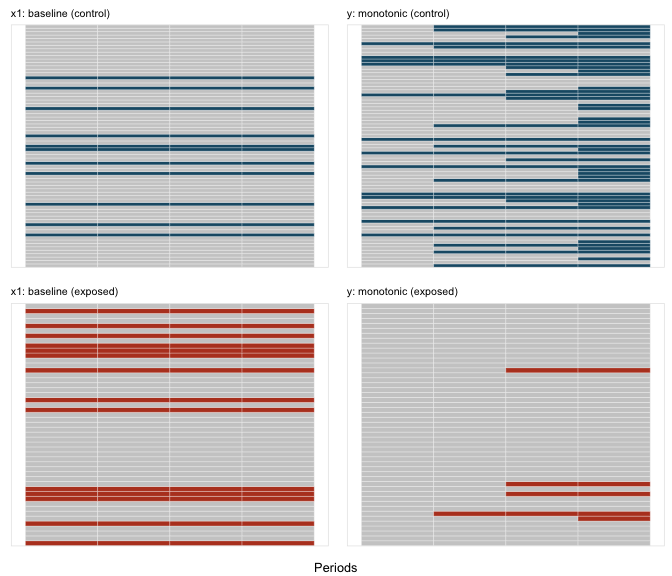# Missing Data

After generating a complete data set, it is possible to generate missing data. defMiss defines the parameters of missingness. genMiss generates a missing data matrix of indicators for each field. Indicators are set to 1 if the data are missing for a subject, 0 otherwise. genObs creates a data set that reflects what would have been observed had data been missing; this is a replicate of the original data set with “NAs” replacing values where missing data has been generated.

By controlling the parameters of missingness, it is possible to represent different missing data mechanisms: (1) missing completely at random (MCAR), where the probability missing data is independent of any covariates, measured or unmeasured, that are associated with the measure, (2) missing at random (MAR), where the probability of subject missing data is a function only of observed covariates that are associated with the measure, and (3) not missing at random (NMAR), where the probability of missing data is related to unmeasured covariates that are associated with the measure.

These possibilities are illustrated with an example. A data set of 1000 observations with three “outcome” measures” x1, x2, and x3 is defined. This data set also includes two independent predictors, m and u that largely determine the value of each outcome (subject to random noise).

def1 <- defData(varname = "m", dist = "binary", formula = 0.5)
def1 <- defData(def1, "u", dist = "binary", formula = 0.5)
def1 <- defData(def1, "x1", dist = "normal", formula = "20*m + 20*u", variance = 2)
def1 <- defData(def1, "x2", dist = "normal", formula = "20*m + 20*u", variance = 2)
def1 <- defData(def1, "x3", dist = "normal", formula = "20*m + 20*u", variance = 2)

dtAct <- genData(1000, def1)

In this example, the missing data mechanism is different for each outcome. As defined below, missingness for x1 is MCAR, since the probability of missing is fixed. Missingness for x2 is MAR, since missingness is a function of m, a measured predictor of x2. And missingness for x3 is NMAR, since the probability of missing is dependent on u, an unmeasured predictor of x3:

defM <- defMiss(varname = "x1", formula = 0.15, logit.link = FALSE)
defM <- defMiss(defM, varname = "x2", formula = ".05 + m * 0.25", logit.link = FALSE)
defM <- defMiss(defM, varname = "x3", formula = ".05 + u * 0.25", logit.link = FALSE)
defM <- defMiss(defM, varname = "u", formula = 1, logit.link = FALSE)  # not observed

set.seed(283726)

missMat <- genMiss(dtAct, defM, idvars = "id")
dtObs <- genObs(dtAct, missMat, idvars = "id")
missMat
##         id x1 x2 x3 u m
##    1:    1  0  0  0 1 0
##    2:    2  0  0  0 1 0
##    3:    3  1  0  0 1 0
##    4:    4  1  0  0 1 0
##    5:    5  1  1  0 1 0
##   ---
##  996:  996  0  0  0 1 0
##  997:  997  1  0  1 1 0
##  998:  998  0  0  1 1 0
##  999:  999  0  0  0 1 0
## 1000: 1000  0  0  0 1 0
dtObs
##         id m  u   x1     x2   x3
##    1:    1 0 NA  2.4 -0.094  1.8
##    2:    2 0 NA 21.0 19.064 21.8
##    3:    3 0 NA   NA -2.126 -2.0
##    4:    4 0 NA   NA  0.635  2.9
##    5:    5 0 NA   NA     NA 17.1
##   ---
##  996:  996 0 NA 17.7 19.671 18.7
##  997:  997 1 NA   NA 40.886   NA
##  998:  998 0 NA 19.8 20.647   NA
##  999:  999 1 NA 20.3 23.645 18.8
## 1000: 1000 0 NA 21.0 20.302 20.0

The impacts of the various data mechanisms on estimation can be seen with a simple calculation of means using both the “true” data set without missing data as a comparison for the “observed” data set. Since x1 is MCAR, the averages for both data sets are roughly equivalent. However, we can see below that estimates for x2 and x3 are biased, as the difference between observed and actual is not close to 0:

# Two functions to calculate means and compare them

rmean <- function(var, digits = 1) {
round(mean(var, na.rm = TRUE), digits)
}

showDif <- function(dt1, dt2, rowName = c("Actual", "Observed", "Difference")) {
dt <- data.frame(rbind(dt1, dt2, dt1 - dt2))
rownames(dt) <- rowName
return(dt)
}

# data.table functionality to estimate means for each data set

meanAct <- dtAct[, .(x1 = rmean(x1), x2 = rmean(x2), x3 = rmean(x3))]
meanObs <- dtObs[, .(x1 = rmean(x1), x2 = rmean(x2), x3 = rmean(x3))]

showDif(meanAct, meanObs)
##              x1   x2   x3
## Actual     19.8 19.8 19.8
## Observed   19.9 18.4 18.0
## Difference -0.1  1.4  1.8

After adjusting for the measured covariate m, the bias for the estimate of the mean of x2 is mitigated, but not for x3, since u is not observed:

meanActm <- dtAct[, .(x1 = rmean(x1), x2 = rmean(x2), x3 = rmean(x3)), keyby = m]
meanObsm <- dtObs[, .(x1 = rmean(x1), x2 = rmean(x2), x3 = rmean(x3)), keyby = m]
# compare observed and actual when m = 0

showDif(meanActm[m == 0, .(x1, x2, x3)], meanObsm[m == 0, .(x1, x2, x3)])
##              x1   x2  x3
## Actual      9.7  9.8 9.7
## Observed    9.8  9.9 8.4
## Difference -0.1 -0.1 1.3
# compare observed and actual when m = 1

showDif(meanActm[m == 1, .(x1, x2, x3)], meanObsm[m == 1, .(x1, x2, x3)])
##              x1   x2   x3
## Actual     30.4 30.4 30.4
## Observed   30.7 31.0 28.6
## Difference -0.3 -0.6  1.8

## Longitudinal data with missingness

Missingness can occur, of course, in the context of longitudinal data. missDef provides two additional arguments that are relevant for these types of data: baseline and monotonic. In the case of variables that are measured at baseline only, a missing value would be reflected throughout the course of the study. In the case where a variable is time-dependent (i.e it is measured at each time point), it is possible to declare missingness to be monotonic. This means that if a value for this field is missing at time t, then values will also be missing at all times T > t as well. The call to genMiss must set repeated to TRUE.

The following two examples describe an outcome variable y that is measured over time, whose value is a function of time and an observed exposure:

# use baseline definitions from the previous example

dtAct <- genData(120, def1)
dtAct <- trtObserve(dtAct, formulas = 0.5, logit.link = FALSE, grpName = "rx")

defLong <- defDataAdd(varname = "y", dist = "normal", formula = "10 + period*2 + 2 * rx",
variance = 2)

dtTime <- addPeriods(dtAct, nPeriods = 4)
dtTime <- addColumns(defLong, dtTime)

In the first case, missingness is not monotonic; a subject might miss a measurement but returns for subsequent measurements:

# missingness for y is not monotonic

defMlong <- defMiss(varname = "x1", formula = 0.2, baseline = TRUE)
defMlong <- defMiss(defMlong, varname = "y", formula = "-1.5 - 1.5 * rx + .25*period",
logit.link = TRUE, baseline = FALSE, monotonic = FALSE)

missMatLong <- genMiss(dtTime, defMlong, idvars = c("id", "rx"), repeated = TRUE,
periodvar = "period")

Here is a conceptual plot that shows the pattern of missingness. Each row represents an individual, and each box represents a time period. A box that is colored reflects missing data; a box colored grey reflects observed. The missingness pattern is shown for two variables x1 and y:In the second case, missingness is monotonic; once a subject misses a measurement for y, there are no subsequent measurements:

# missingness for y is monotonic

defMlong <- defMiss(varname = "x1", formula = 0.2, baseline = TRUE)
defMlong <- defMiss(defMlong, varname = "y", formula = "-1.8 - 1.5 * rx + .25*period",
logit.link = TRUE, baseline = FALSE, monotonic = TRUE)

missMatLong <- genMiss(dtTime, defMlong, idvars = c("id", "rx"), repeated = TRUE,
periodvar = "period")